Next: Type-II Superconductors and the Vortex State Up: Magnetic Properties of Conventional Superconductors Previous: The Clean and Dirty Limits

## BCS Theory for Conventional Superconductors

In 1957 the underlying microscopic theory of superconductivity in metals was unveiled by J. Bardeen, L.N. Cooper and J.R. Schrieffer , in the now famous BCS theory. In normal metals, the situation is well described by free electron theory, where the electrons behave as free particles and the metallic ions play a limited role in conductivity. BCS theory outlines how in the presence of an attractive interaction between electrons (Cooper pairs), the normal state of an otherwise free electron gas becomes unstable to the formation of a coherent many-body ground state. The mechanism behind the weak attractive force binding the Cooper pairs was actually first suggested by Herbert Frölich . He proposed that the same mechanism responsible for much of the electrical resistivity in metals (i.e. the interaction of conduction electrons with lattice vibrations) leads to a state of superconductivity. This hypothesis of an electron-phonon interaction was born out of experiments which found that the critical temperature Tcvaried with isotopic mass. In simple terms, an electron interacts with the lattice by virtue of the Coulomb attraction it feels for the metallic ions. The result is a deformation of the lattice (i.e. a phonon). A second electron in the vicinity of the deformed lattice correspondingly lowers its energy, resulting in an electron-electron attraction via a phonon. Viewed in this context, the superconducting order parameter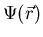from GL-theory can be interpreted as a one-particle wave function describing the position of the center of mass of a Cooper pair .

Despite being an extremely weak attraction, bound pairs form in part because of the presence of a Fermi sea of additional electrons. As a result of the Pauli exclusion principle, electrons that would prefer to be in a state of lower kinetic energy cannot populate these states because they are already occupied by other electrons. Thus a Fermi sea is required to ensure the formation of bound pairs of electrons; otherwise an isolated pair of electrons would just repel one another as a result of the Coulomb force between them. The Fermi sea itself is comprised of other distinct bound pairs of electrons. It follows that each electron is a member of both a Cooper pair and of the Fermi sea which is necessary for the formation of all Cooper pairs. The force of attraction between the electrons which comprise a Cooper pair has a range equivalent to the coherence length. It should be noted that the separation between electrons in a Cooper pair (and thus the correlation length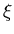), for a type-I superconductor, is large enough that millions of other pairs have their centers of mass positioned between them. It is then assumed that the occupancy of a bound pair is instantaneous and uncorrelated with the occupancy of other bound pairs at an instant in time . Armed with knowledge of the fundamental particles responsible for superconductivity (i.e. Cooper pairs), the substitutions q=2e and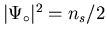immediately transform the Ginzburg-Landau result for the penetration depth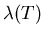[i.e. Eq. (2.6)] into the result predicted by London theory [i.e. Eq. (2.1)].

One of the most remarkable features emerging from BCS theory, is the existence of an energy gap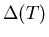between the BCS ground state and the first excited state. It is the minimum energy required to create a single-electron (hole) excitation from the superconducting ground state. Thus the binding energy of a Cooper pair is two times the energy gap. BCS theory estimates the zero-temperature energy gap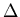(0) as :(17)

and near the critical temperature Tc,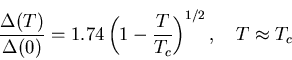(18)

so that the energy gap approaches zero continuously as. Superconductors which obey Eq. (2.17) are considered to be weakly-coupled, in reference to the weak interaction energy between electrons in a Cooper pair. Furthermore, the wave functions corresponding to electron pairs (Cooper pairs), are spatially symmetric like an atomic s-orbital with angular momentum L=0. That is to say, the wave function of a pair is unchanged if the positions of the electrons are exchanged. This immediately implies that the spin part of the wave function is antisymmetric in accordance with the Pauli exclusion principle. In particular, the electron pairs are in a spin-singlet state S=0with antiparallel spins. The pairing mechanism in a conventional superconductor is thus appropriately called, s-wave spin-singlet. The energy gap of an s-wave superconductor is finite over the entire Fermi surface. Under ideal circumstances, the magnitude of the gap is the same at all points on the Fermi surface. BCS theory assumes the Fermi surface is spherical (see Fig. 2.3). More realistically however, the energy gap reflects the symmetry of the crystal under consideration . For a conventional s-wave superconductor with a weak-coupling ratio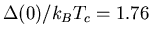, BCS theory predicts: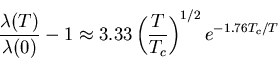(19)

for small T (i.e. T < 0.5Tc) [17,29]. At low temperatures, the energy gap is virtually independent of temperature and much larger than the thermal energy kBT. The probability of exciting a single electron with energy Ek is then proportional to the Boltzmann factor e-Ek/kBT. The maximum value of this probability is proportional to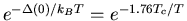, which is the exponential factor appearing in Eq. (2.19). Therefore in conventional superconductors,shows an exponential decrease at low temperatures.

If the value of the energy gap is not constant over the entire Fermi surface, then the minimum value of the gap determines the density of quasiparticle excitations at these low temperatures. Hence the topology of the energy gap is crucial in deciding the low-temperature behaviour of. If the sample under investigation is riddled with impurities, then there will exist a broad range of transition temperatures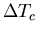. Equations (2.17) and (2.18) suggest that one should anticipate a corresponding distribution of gap energies in such materials.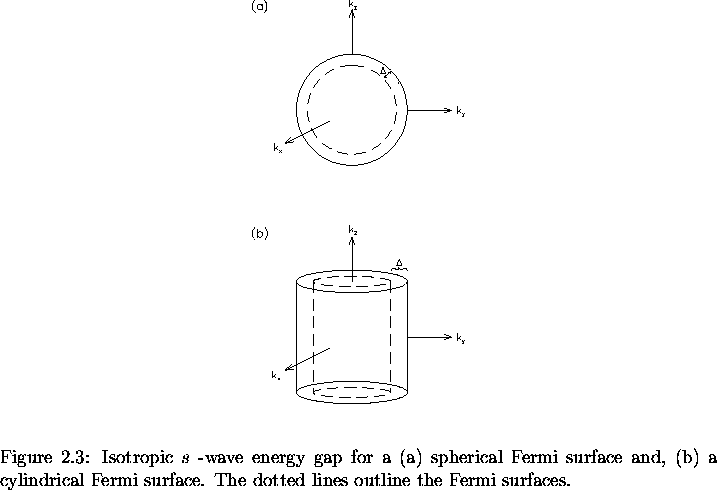Some systems (e.g. lead, mercury) produce experimental results which deviate substantially from the BCS results . These materials are more appropriately described by strong-coupling theory where the coupling ratio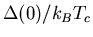is greater than the BCS prediction of 1.76. Under certain conditions superconductivity can occur without an energy gap in some materials. Tunneling experiments on superconductors with specific concentrations of paramagnetic impurities show this to be possible . Theories exist which explain such anomalies, and the nature of the gap as we will soon see is a vital property to be considered in any theory describing superconductivity in the high-Tc compounds.Next: Type-II Superconductors and the Vortex State Up: Magnetic Properties of Conventional Superconductors Previous: The Clean and Dirty Limits
Jess H. Brewer
2001-09-28